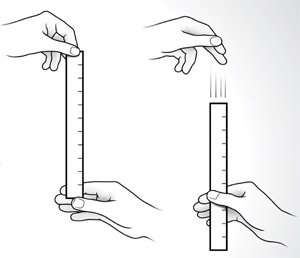## Testing Reaction Times with Excel

Suppose you are interested in testing whether reaction times of students generally agree or are different from person to person. As a step in this direction, conduct the experiment detailed below, which uses hypothesis testing to compare the mean reaction times of three students.

1. Collect Data Using the Ruler Drop Test
To perform the "Ruler Drop Test", first obtain a 30 cm ruler. Then, have one student hold the ruler vertically with lower numbers at the bottom. Another student places his/her thumb and index finger at (but not touching) the bottom of the ruler, ready to catch it. The first student drops the ruler (without warning) and the second student catches it. Record the distance in millimeters the ruler dropped before it was caught. (Each student should practice a few times before you start recording data.)Collect at least 30 “drop distances” each from 3 students. Try to ensure that the conditions of the test are identical for all of the students. Enter your data into an Excel spreadsheet.

2. Calculate Reaction Times
You probably learned in a math or a physics class that the distance an object drops is proportional to the square of the time it spends falling. Specifically, $d=(1/2)gt^2$, where $g=9.81$ $\textrm{m}/\textrm{s}^2$. Use this fact to convert your distances to times in milliseconds in your Excel spreadsheet.

3. Check Assumptions
Determine whether the ANOVA test is appropriate for testing the means of the 3 resulting data sets by doing the following in an Excel worksheet:

1. Construct a frequency distribution and histogram for each sample.
2. Test for and remove outliers using the IQR definition for outliers.
3. Test the skewness of the remaining data using the Pearson’s skewness index.
4. If normality conditions are met, perform pairwise tests of the variances.

4. Perform an Appropriate Test
Use the parametric ANOVA test if all assumptions are met, otherwise use the non-parametric Kruskal-Wallis test (do not do both tests). Use Excel to calculate the appropriate test statistics from their formulas (don't use any predefined tests in Excel to calculate these test statistics).

Note: an ANOVA test should include an ANOVA table that you calculate from the formulas. Also, use either a $p$-value or a critical value calculated using Excel functions. You may find the following functions useful to these ends: RANK.AVG, F.DIST, F.INV, CHISQ.DIST, CHISQ.INV.